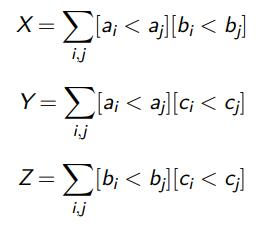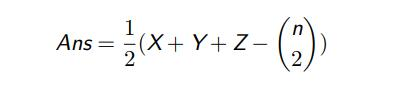# 基础分治练习题

#### 1.2 输入格式

``````const int N = 2e6+5;
unsigned int SA,SB,SC;int n,a[N],b[N],c[N];
unsigned int rd()
{
SA^=SA<<16;SA^=SA>>5;SA^=SA<<1;
unsigned int t=SA;SA=SB;SB=SC;SC^=t^SA;return SC;
}
void gen(int *P)
{
for (int i=1;i<=n;++i) P[i]=i;
for (int i=1;i<=n;++i) swap(P[i],P[1+rd()%n]);
}
int main()
{
scanf("%d%u%u%u",&n,&SA,&SB,&SC);
gen(a);gen(b);gen(c);return 0;
}``````

#### 1.4 样例 1 输入

``````5
233 666 667``````

#### 1.5 样例 1 输出

``4``

#### 1.7 样例 2 输入

``````100000
123 456 789``````

#### 1.8 样例 2 输出

``1258889897``

#### 1.10 提示